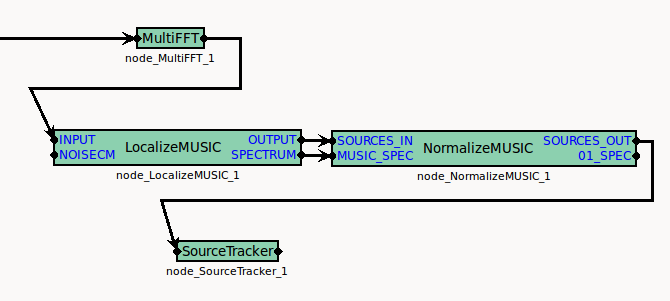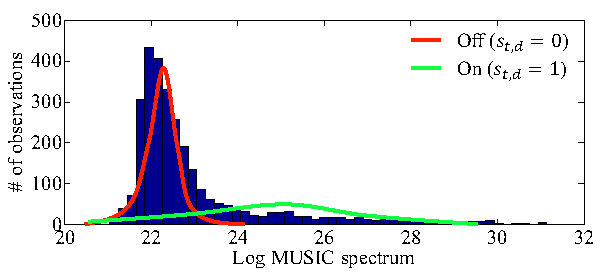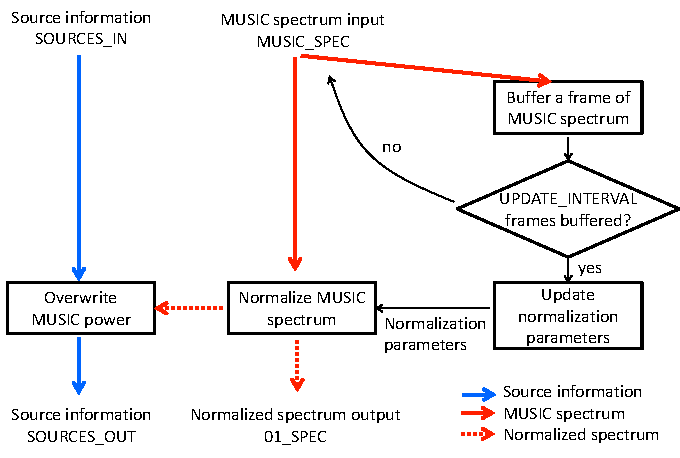## 6.2.16 NormalizeMUSIC

### 6.2.16.1 Outline of the node

This module normalizes the MUSIC spectrum calculated by LocalizeMUSIC into the range $[0~ 1]$ so as to stabilize the sound source detection by the thresholding in SourceTracker .

None.

### 6.2.16.3 Usage

When to use

Sound source localization and detection functions in HARK are achieved by applying a threshold on the MUSIC spectra for each discretized time and direction1. This module helps you to determine an appropriate threshold in SourceTracker by normalizing the MUSIC spectra calculated by LocalizeMUSIC instead of by directly applying a threshold on the MUSIC spectra. By using this module and setting the threshold in SourceTracker at a value close to 1, e.g. 0.95, the source localization performance becomes stable.

This module internally calculates the normalization parameters and normalizes the MUSIC spectrum values between $[0~ 1]$. The estimation of normalization parameteres are carried out at a certain interval using the observed MUSIC spectrum frames. In this estimation, the distributions corresponding to the presence or absence of sound sources. The MUSIC spectrum is normalized for each time frame by a particle filter using the estimated normalization parameters.

Typical connection

Figure 6.41 shows a typical usage of NormalizeMUSIC module. In Fig. 6.41, the outputs of LocalizeMUSIC (OUTPUT: source locations and corresponding MUSIC spectrum values, SPECTRUM: MUSIC spectrum for each direction) are connected to the corresponding inputs of NormalizeMUSIC module. SOURCES_OUT of NormalizeMUSIC can be connected with SourceTracker in the same way as the output of LocalizeMUSIC .Figure 6.41: Typical usage of NormalizeMUSIC

### 6.2.16.4 Input-output and property of the node

Table 6.33: Parameter list of NormalizeMUSIC
 Parameter name Type Default value Unit Description SHOW_ALL_PARAMETERS false Show or hide the parameters except INITIAL_THRESHOLD. INITIAL_THRESHOLD 30 An approximate boundary on MUSIC spectra where a sound source exists or not. ACTIVE_PROP 0.05 A threshold whether to udpate the normalization parameters. UPDATE_INTERVAL 10 The interval of updating the normalization parameters, and the number of frames used for the process. PRIOR_WEIGHT 0.02 Regularization parameter for the calculation of the normalization parameters. MAX_SOURCE_NUM 2 The maximum number of sources that each particle can assume in the particle filter below. PARTICLE_NUM 100 The number of particles used by the particle filter that normalizes the MUSIC spectra. LOCAL_PEAK_MARGIN 0 A margin of MUSIC spectrum between adjacent directions for the detection of local peaks in the MUSIC spectrum.

Inputs

SOURCES_IN

: Vector<ObjectRef> type. Connected with OUTPUT node of LocalizeMUSIC . This contains source information (location and MUSIC spectrum value).

MUSIC_SPEC

: Vector<float> type. Connected with SPECTRUM node of LocalizeMUSIC . This contains the MUSIC spectrum value for each direction.

Outputs

SOURCES_OUT

: Vector<ObjectRef> type. This contains the same source information as SOURCES_IN except that the MUSIC spectrum value of each source is replaced with the normalized value in $[0~ 1]$.

01_SPEC

: Vector<float> type. Normalized values of the input node MUSIC_SPEC.

Parameters

SHOW_ALL_PARAMETERS

: bool type, set falseby default。・If set true, all parameters are displayed. In many cases, parameters other than INITIAL_THRESHOLD require no modification from the default values.

INITIAL_THRESHOLD

: float type, set 30 by default. This value is used in two ways: (1) used as a prior belief of the boundary on the MUSIC spectrum between the presence and absence of sound source, and (2) used to determine whether to carry out the first estimation of the normalization parameters.

ACTIVE_PROP

: float type, set $0.05$ by default. This is a threshold to determine whether to update the normalization parameters when UPDATE_INTERVAL frames of MUSIC spectra are accumulated. Let $T$ be the number of frames of MUSIC spectra and $D$ be the number of directions, that is $TD$ MUSIC values in total, and $\theta$ denote ACTIVE_PROP. If we have more time-direction points than $\theta TD$ in the MUSIC spectra with a larger value than INITIAL_THRESHOLD, the normalization parameters are updated.

UPDATE_INTERVAL

: int type, set 10 by default. The number of frames used to update the normalization parameters. This value controls the interval of the updates. By default, HARK system is configured as follows. The multichannel audio signal is sampled at 16000 (Hz). In MultiFFT , the short-time Fourirer transform is carried out with 160 (pt) shift, that is 0.01 (sec). LocalizeMUSIC module calculates the MUSIC spectrum every 50 frames, i.e., 0.5 (sec). Therefore, if UPDATE_INTERVAL is 10, the normalization parameters are calculated with the MUSIC spectra for 5 (sec).

PRIOR_WEIGHT

: float type. This is a regularization parameter to stabilize the estimation of the normalization parameters. Specific explanation is provided in Technical details, and recommended configuration is given in Troubleshooting below, respectively.

MAX_SOURCE_NUM

: int type. The maximum source number that each particle can hypothesize in the particle filter for the MUSIC spectrum normalization. Regardless of the actual number of sound sources, setting this parameter at $1–3$ produces good results.

PARTICLE_NUM

: int type, set 100 by default. The number of particle used in a particle filter to normalize the MUSIC spectrum in each time frame. Empirically, 100 is a sufficiently large number when we have 72 directions ($5^\circ$ resolution on the azimuth plane). If you handle more directions (for example, multiple elevations like $72 \times 30$ directions), more particles may be necessary.

LOCAL_PEAK_MARGIN

: float type. In the particle filter for the MUSIC spectrum normalization, each particle can hypothesize an existence of sound sources at local peaks of the observed MUSIC spectrum. This value is the margin allowed to take the local peaks. Setting a too large value for this parameter may risk false detections of sound sources.

### 6.2.16.5 Details

Technical details: The interested readers can refer to the paper in 6.2.16.6 Reference below. In the paper, the normalization parameter estimation corresponds to the estimation of the posterior distribution of VB-HMM (variational Bayes hidden Markov model), and the MUSIC spectrum normalization corresponds to the online inference using a particle filter.Figure 6.42: Estimation of normalization parameters from observed MUSIC spectra

Roughly speaking, this module fits to Gaussian distributions, sound presence in green in Fig. 6.42 and sound absence in red, to the observed MUSIC spectra (blue histogram in Fig. 6.42).

Here we provide how the variables used in the paper correspond to the parameters of this module. The input of the VB-HMM for the normalization parameter estimation is MUSIC spectra with $T$ time frames and $D$ directions. $T$ is specified by UPDATE_INTERVAL and $D$ is determined in LocalizeMUSIC module. VB-HMM uses some hyperparameters $\alpha _{0}, \beta _{0}, m_0, a_0$, and $b_0$. $\alpha _0 a_0$, and $b_0$ are set at $1$ in the same way as the reference. $m_0$ is the INITIAL_THRESHOLD, and $\beta _0$ is set at $TD\varepsilon$ provided that PRIOR_WEIGHT is $\varepsilon$.

Flowchart:Figure 6.43: Flowchart of NormalizeMUSIC

Figure 6.43 illustrates the flowchart of NormalizeMUSIC module. Blue lines are a flow of source information from SOURCES_IN to SOURCES_OUT. Red solid lines are the observed MUSIC spectrum and red dotted lines are the normalized MUSIC spectrum. Two processes are carried out for each time frame: (1) Normalization of MUSIC spectrum using the latest normalization parameters (middle column). (2) Replacement of the MUSIC spectrum value in the source information with the normalized value (left column). SourceTracker module that follows NormalizeMUSIC module refers to the MUSIC spectrum value in the source information to detect and localize the sound sources.

The right column is the update of normalization parameters. When the following two conditions are satisfied, the normalization parameters are udpated.

1. UPDATE_INTERVAL frames of MUSIC spectra are accumulated, and

2. the proportion of time-direction points with sound existence exceeds ACTIVE_PROP.

When 1. is satisfied, whether condition 2. is satisfied is examined using the observed MUSIC spectra $x_{t,d}$ with $T$ frames and $D$ directions. Let $\theta$ be ACTIVE_PROP.
First update: In case no normalization parameter estimation has been carried out since the program was started, the first estimation of the normalization parameters are carried out if the number of time-direction points $x_{t,d}$ more than INITIAL_THRESHOLD exceeds $\theta TD$.
Updates afterwards: If the summation of the normalized MUSIC spectrum values exceeds $\theta TD$, the normalization parameters are updated using the latest observation of MUSIC spectra.

Troubleshooting: We outline how to configure the parameters when something is wrong with the localization and detection of sound sources.

Visualize MUSIC spectrum: Verify that the values of MUSIC spectrum are low in the absence of sound sources and high in the presence. You can obtain the MUSIC spectrum values by setting DEBUG parameter at truein LocalizeMUSIC module. Then, the values are streamed into the standard output. You can visualize these values by using an appropriate tool such as python + matplotlib or matlab. You can find a similar topic in “3.3 Sound source localization fails” of HARK cookbook. If there seems to be something wrong with the calculation of MUSIC spectra, see “8 Sound source localization” of HARK cookbook. The calculation of MUSIC spectra is sometimes stabilized by setting NUM_SOURCE parameter at 1 and LOWER_BOUND_FREQUENCY parameter at 1000 (Hz) of LocalizeMUSIC .

Try first: Set ACTIVE_PROP and PRIOR_WEIGHT at 0. Try a low value for INITIAL_THRESHOLD (for example 20). Set THRESH parameter at 0.95 of SourceTracker module connected with SOURCES_OUT of NormalizeMUSIC module. If nothing is localized, the calculation of MUSIC spectra is likely to be wrong. If too many sounds are detected, adjust INITIAL_THREHSHOLD by increasing at 5 intervals (e.g., 20 $\rightarrow$ 25 $\rightarrow$ 30).

Too many false detections: Possible reasons are (1) MUSIC spectrum value is high in the absence of a sound source and (2) the mean value of the distribution for sound presence in Fig. 6.42 is too low. In case of (1), we can try the MUSIC algorithm that uses noise correlation matrix (see LocalizeMUSIC for details), or increasing LOWER_BOUND_FREQUENCY of LocalizeMUSIC to 800–1000 (Hz). In case of (2), we can try increasing INITIAL_THRESHOLD (for example, from 30 to 35), or PRIOR_WEIGHT (for example, set around 0.05–0.1).

Nothing is detected: Possible reasons are (1) MUSIC spectrum value is low even in the presence of a sound source and (2) INITIAL_THRESHOLD is too large. In case of (1), we have to adjust the parameters of LocalizeMUSIC . Set NUM_SOURCES at the actual number of sources, or try a larger value like $M-1$ or $M-2$, where $M$ is the number of microphones. Specify LOWER_BOUND_FREQUENCY and UPPER_BOUND_FREQUENCY to meet the frequency range of the target sound. In case of (2), decrease INITIAL_THRESHOLD.

### 6.2.16.6 Reference

• Takuma Otsuka, Kazuhiro Nakadai, Tetsuya Ogata, Hiroshi G. Okuno: Bayesian Extension of MUSIC for Sound Source Localization and Tracking, Proceedings of International Conference on Spoken Language Processing (Interspeech 2011), pp.3109-3112. 2

Footnotes

1. For instance, 10 ms time resolution and $5^\circ$ directional resolution
2. http://winnie.kuis.kyoto-u.ac.jp/members/okuno/Public/Interspeech2011-Otsuka.pdf